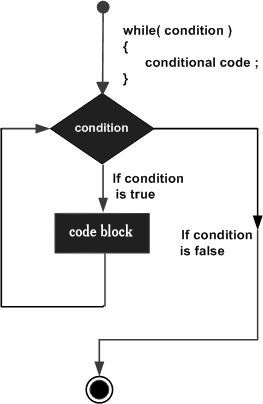# Tcl - While Loop

A while loop statement in Tcl language repeatedly executes a target statement as long as a given condition is true.

## Syntax

The syntax of a while loop in Tcl language is −

```while {condition} {
statement(s)
}
```

Here, statement(s) may be a single statement or a block of statements. The condition may be any expression, and true is any nonzero value. The loop iterates while the condition is true.

When the condition becomes false, program control passes to the line immediately following the loop.

## Flow DiagramThe point to note about the while loop is that the loop might not ever run. When the condition is tested and the result is false, the loop body will be skipped and the first statement after the while loop will be executed.

## Example

```#!/usr/bin/tclsh

set a 10

#while loop execution
while { \$a < 20 } {
puts "value of a: \$a"
incr a
}
```

When the above code is compiled and executed, it produces the following result −

```value of a: 10
value of a: 11
value of a: 12
value of a: 13
value of a: 14
value of a: 15
value of a: 16
value of a: 17
value of a: 18
value of a: 19
```
tcl_loops.htm